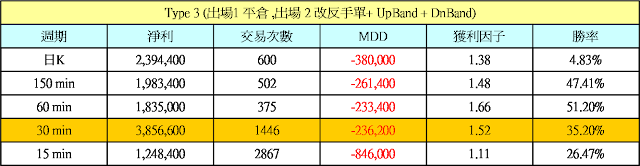## 2013年12月6日 星期五

### 艾達透視指標 Elder Ray 交易模型 Part 3 (程式碼)if Type= 3 then Begin
Plot1(BullPower,"Bull",iff(BullPower >= 0,Magenta,Yellow),Black,1) ;
Plot2(BearPower,"Bear",iff(BearPower >= 0,White,Green),Black,1) ;
Plot3(UpBand,"UpBand") ;
Plot4(-DnBand,"DnBand") ;

{ for MultiChart
Plot5(HighBand,"HighBand") ;
Plot6(-LowBand,"LowBand") ; }
end;input:HLRange1(50),HLRange2(250),TscoreGap(6);
Vars:PFpoint(0),LossPoint(0),HL(0) ,TTXN_Score(0),Zscore1(0),Tscore1(0),TTXN(0);

if DataCompression > 1 then Begin
HL = High-Low ;
Condition1 = Close > Open and Open > Close ;
Condition2 = Close < Open and Open < Close ;
end else Begin
HL = HighD(1)-LowD(1) ;
Condition1 = Close > OpenD(0) and OpenD(0) > CloseD(1) ;
Condition2 = Close < OpenD(0) and OpenD(0) < CloseD(1) ;
end;

MP = MarketPosition ;

if DAYofMonth(Date) > 14 and DAYofMonth(Date) < 22 and DAYofWeek(Date)= 3 then isBalanceDay = True else isBalanceDay =False ;

HighOsc = High - Xaverage(High,BaseLen) ;
LowOsc = Low - Xaverage(Low,BaseLen) ;
CloseAvg = Xaverage(Close,RefLen) ;

Condition3 = HL > HLRange1 and HL < HLRange2 ;
Condition4 = Highest(High,RefLen)-Lowest(Low,RefLen) < HLRange2 ;

if date <> date then Begin
Value92 = Close of Data3 ;
if StdDev(Value92,15) <> 0 then
Zscore1 = (Value92  - Average(Value92,15))/StdDev(Value92,15);
TScore1 = 10*Zscore1 + 50 ;

{ TTXN }
if StdDev(Close,15) <> 0 then
TTXN_Score = (Close- Average(Close,15))/StdDev(Close,15);
TTXN = 10*TTXN_score + 50 ;
Condition5 = TTXN > Tscore1 and TTXN > HLRange1 ;
Condition6 = TTXN < TScore1 and TTXN < HLRange2 ;
Condition7 = Tscore1 > HLRange1 and AbsValue(TTXN-Tscore1) < TscoreGap;
Condition8 = Tscore1 < HLRange2 and AbsValue(TTXN-Tscore1) < TscoreGap;
Condition9 = TTXN > HLRange1 ;
Condition10 = TTXN < HLRange2 ;
end;

Value9 = Dayofweek(date) ;
Condition11 = ( Value9 = 1 ) ;
Condition12 = (Value9 = 5 or Value9 = 4) ;
Condition13 = Close > MaxList(HighD(1),HighD(2),HighD(3)) ;
Condition14 = Close < MinList(LowD(1),LowD(2)) ;
BuySetup = CloseAvg > CloseAvg and Close > CloseAvg and LowOsc < -DnBand ;
SellSetup = CloseAvg < CloseAvg and Close < CloseAvg and HighOsc > UpBand ;

{ 30 min K
input:HLRange1(30),HLRange2(30),TscoreGap(9);}

{主程式碼 }
if HighOsc > UpBand and LowOsc > UpBand and HighOsc > LowOsc
and Condition7 then Buy ("HB") next bar at Market ;
if HighOsc < -DnBand and LowOsc < -DnBand and HighOsc < LowOsc
and Condition8 then Sell ("LS") next bar at Market ;

setProfitTarget(PFPoint*BigPointValue) ;
setstoploss(LossPoint*BigPointValue) ;

if IsBalanceDay then SetExitonClose ;MagicQS018_1

#### 6 則留言:

1.版主您好
又再次打擾你,想請問一下 Value92 = Close of Data3 的DATA3是用甚麼資料呢?

謝謝

2.您好:請參考版上分析師的領先指標系列文章介紹

3.您好~我是剛接觸程式交易不久的新手，想請問一下avgprice是什麼意思?

4.avgprice = (Open+High+Low+Cloe)/4

5.avgprice = (Open+High+Low+Cloe)/4

6.非常感謝您的回答